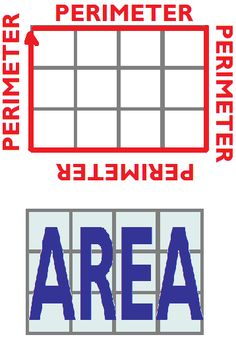# Area of 2D Shapes

##BIG IDEAS:

(taken from “Big Ideas by Dr. Small”):

1. Familiarity with known benchmark measurements can help you estimate and calculate other measurements.
2. The unit chosen for a measurement affects the numerical value of the measurement; if you use a bigger unit, fewer units are required.
3. The length of an object is a one-dimensional attribute. Length can be the measurement of a single measure of an object or a combined linear measure, like perimeter.
4. The area of an object is a two-dimensional attribute. Area can be a single measure of a 2-D shape on an object or a combined measure of a 3-D shape, like surface area.

## STUDENT LEARNING GOALS:

• I can estimate the lengths of objects using known benchmarks.
• I can convert between different metric units.
• I can determine the perimeter of polygons.
• I can use a formula to determine the area of basic shapes, including trapezoids.
• I can determine the area of composite shapes.

## CURRICULUM EXPECTATIONS:

• Solve problems that require conversion between metric units of measure (e.g., millimetres and centimetres, grams and kilograms, millilitres and litres)
• Solve problems that require conversion between metric units of area (i.e., square centimetres, square metres) (Sample problem: What is the ratio of the number of square metres to the number of square centimetres for a given area? Use this ratio to convert 6.25 m2 to square centimetres.);
• Determine, through investigation using a variety of tools (e.g., concrete materials, dynamic geometry software) and strategies, the relationship for calculating the area of a trapezoid, and generalize to develop the formula [i.e., Area = (sum of lengths of parallel sides x height) ÷ 2] (Sample problem: Determine the relationship between the area of a parallelogram and the area of a trapezoid by composing a parallelogram from congruent trapezoids.);
• Solve problems involving the estimation and calculation of the area of a trapezoid;
• Estimate and calculate the area of composite two-dimensional shapes by decomposing into shapes with known area relationships (e.g., rectangle, parallelogram, triangle) (Sample problem: Decompose a pentagon into shapes with known area relationships to find the area of the pentagon.);
• Research and report on real-life applications of area measurements (e.g., building a skateboard; painting a room);